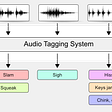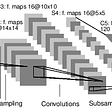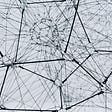# Understanding Markov Localization

Imagine you are traveling in a car on a known route and you fall asleep. When you wake up, you have no idea where in the city you are. You observe the surrounding to see the known houses, shops, boards, and signs and after a second or two, you understand your location just by analyzing your surrounding. That's what localization does!

After reading numerous articles on localization, I finally got an overview of the concept. But, I was not clear about how to exactly reflect this in my code: how to actually calculate the probability terms in the equations. I will try explaining the concept and the code is the simplest way possible.

First, let's go through a brief introduction of Markov’s localization.

Markov’s localization is an application of Bayesian filter algorithm, which is a fundamental and core concept in probabilistic robotics. The Bayesian filter algorithm is a two-step process.

1. Prediction step:
bel’(xₜ) = Σp(xₜ | uₜ , xₜ₋₁)* bel(xₜ₋₁)
2. Update step.
bel(xₜ) = η * p(zₜ | xₜ) * bel’(xₜ)

(I know, the equations look scary at first, but don’t get intimidated!)

The prediction step evaluates the belief of state xₜ(robot’s state at time t) using the belief at time t-1(xₜ₋₁) and control input. For example, I am standing at x=0 at time t-1 (i.e. xₜ₋₁ = 0), and someone pushes me with an intent to make me stand at x=1 at time t(i.e.xₜ = 1). I can say that the control input is something proportional to Δx (let’s say k*Δx with k=1) i.e. 1 – 0. Thus, I can say that xₜ = xₜ₋₁ + Δx. If the position at xₜ₋₁, i.e. my last position, is known, then from the equation above, I can predict the position at the instant t.

The word ‘prediction’ gets automatically associated with the probability. Thus, for a model, there will be a probability distribution function, which is assumed to be a Gaussian function.(I will explain in later part how to calculate the same). Hence, p(xₜ | uₜ , xₜ₋₁) in (1) is a probability distribution function. The belief bel’(xₜ ) that the robot assigns to state xₜ is obtained by the summation of the product of two distributions: Belief assigned to prior state xₜ₋₁ and the PDF based on the control input.

The update or the measurement step is quite interesting. Generally, given a sensor reading, we can predict the actual value based on the error in sensor measurement. Here in the measurement step, we predict the sensor value from the actual state. We evaluate the probability that the measurement z may have been observed, given the state xₜ. Multiplying the term with bel’(xₜ) and normalizing it with factor η, gives us bel(xₜ), which is the probability distribution of robot in state x at time t (xₜ).

Please go through Chapter 2 of PROBABILISTIC ROBOTICS by Sebastian Thurn, Wolfram Burgard, and Dieter Fox to understand Bayesian Filter in details. The book can be downloaded from here.

Now, let me explain with an example. There is a robot named Onro (One-dimensional Robot). It has a sensor which measures the object distance in only one dimension. There is a unidimensional environment and we have a 1D map of that environment. The map looks something like this.

The map features(blue crosses) are at the markings as shown and the number signifies the distance of those features with respect to the map frame(map origin). So, we can say the cross 1 is 9 meter from map origin, cross 2 is 15 meter from map origin and so on. Onro has a sensor which can measure the relative distance of the object. Onro also has knowledge about the control inputs it is generating in each step. Now let’s put this in code!

All this data and information are stored in .csv file. The first step is to read and store the data in a suitable data structure. Refer the functions ReadMeasurementData and ReadMapData in help_functions.cpp(code link in later part). Now the confusing part begins...

Before that, a quick explanation of how the belief(bel(x)) is represented here. Any bel function is a normal distribution function(bell-shaped). It is discretized with 100 points(refer figure below) as x varies from 0 to 100, with an interval 1. Note that, features are located at only a few points. For example 9, 15, etc. out of all the hundred points.

For simplification, the control input is assumed to be 1, i.e. Δx = 1 for all the cases. Also, the covariance is assumed as 1.

Onro is set free in the mapped environment. Initially, it has no idea where on the map it is located. Hence, we assign equal probability to all the possible places. Note that, the summation of probabilities at all points(100 in our case), must be equal to 1, hence, normalize the distribution after each iteration.

Next, it got a control input which is equal to 1. Now let's look at it in this way. Onro is at point 5. In mathematical terms, the probability that Onro is at 5 is 0.8(assumed). After applying a control input of 1, Onro should reach 6.

xₜ = xₜ₋₁ + Δx(already discussed)

xₜ -xₜ₋₁ = Δx

The probability of Onro to be at 6 will be maximum. i.e., the probability that( xₜ -xₜ₋₁) value to be 1 will be maximum. The probability that Onro is at 7 will be lesser, i.e., the probability of getting the value of (xₜ -xₜ₋₁) as 2 will be lesser. Similarly, the probability that the value of (xₜ -xₜ₋₁) is 3,4,5 and so on, will be in decreasing order. Imagine this for all 100 points. The probability that Onro is at 100 will be 0. We can get a normal distribution for (xₜ -xₜ₋₁) with mean as 1(most likely value for (xₜ -xₜ₋₁)) and co-variance as 1(assumed as 1). Putting it in other words, for a particular xₜ₋₁, we can plot a normal distribution centered at xₜ₋₁+Δx.(Think think!). This yields my p( xₜ | uₜ, xₜ₋₁) term in step 1 on the Bayesian filter. Multiply it with the previous belief of x and perform the summation of the product for 100 iterations to get posterior control belief(bel’( xₜ)).

Now, we will update the posterior control probability further in the measurement step. We will be using the sensor reading and map data for calculating a posterior observation probability. For comparison of any quantities, the crucial step is to get them in the same unit and frame. The features in the map are in map frame, while the sensor data is in the inertial frame(Onro’s frame). Refer lines 10 to 29 in the code below converts map features in Onro’s frame.

As per the update step of Baye’s filter, we predict the sensor value(Observation) from the robot’s state. Hence, for my normal distribution, we will find the probability of getting the specific sensor reading, given the map feature in Onro’s frame(Confused? Detailed explanation after the code).

We got our posterior control probability and posterior observation probability. Multiply the both to get a belief for the state at time t.

After a few iterations, we will obtain a bell function with its mean at our robot’s state. hence, we can say that we are localized (:D). I recommend plotting graphs after each iteration for better understanding.

The original code can be downloaded from this git repo. I made a few modifications and made a ROS package for further extension. Refer this to access the modified code. After successful execution of code, text files will be generated for prediction and observation steps. This data can be used for plotting the PDFs.

I tried to explain Markov’s Localisation concept in a very simple way. This is my first tech article and thus can be prone to errors. For any queries, suggestions or feedback, please contact me on LinkedIn(Sakshi Kakde). I will be happy to hear from you! :)

--

--

--

## More from Sakshi Kakde

Aspiring Roboticist. ❤️ to explore

Love podcasts or audiobooks? Learn on the go with our new app.

## Regularization for machine learning in terms a child could understand.## Freesound Audio Tagging — Recognizing Sounds of Various Natures## Multi-Class Image Classification with TensorFlow## Neural Network Tuning with TensorFlow## DeepSign : Indian Sign Language (Phase 0)## Self-Supervised Learning in Computer Vision — MoCo V1 and Barlow Twins## Monitoring urban development from space## End to End Machine Learning with H2O.ai## Sakshi Kakde

Aspiring Roboticist. ❤️ to explore

## Transfer Learning to predict student performance## Performing Speech-to-Text Recognition with OpenVINO™## Global average pooling